# Example: Parkinson’s disease

library(multinma)
options(mc.cores = parallel::detectCores())

This vignette describes the analysis of data on the mean off-time reduction in patients given dopamine agonists as adjunct therapy in Parkinson’s disease, in a network of 7 trials of 4 active drugs plus placebo (Dias et al. 2011). The data are available in this package as parkinsons:

head(parkinsons)
#>   studyn trtn     y    se   n  diff se_diff
#> 1      1    1 -1.22 0.504  54    NA   0.504
#> 2      1    3 -1.53 0.439  95 -0.31   0.668
#> 3      2    1 -0.70 0.282 172    NA   0.282
#> 4      2    2 -2.40 0.258 173 -1.70   0.382
#> 5      3    1 -0.30 0.505  76    NA   0.505
#> 6      3    2 -2.60 0.510  71 -2.30   0.718

We consider analysing these data in three separate ways:

1. Using arm-based data (means y and corresponding standard errors se);
2. Using contrast-based data (mean differences diff and corresponding standard errors se_diff);
3. A combination of the two, where some studies contribute arm-based data, and other contribute contrast-based data.

Note: In this case, with Normal likelihoods for both arms and contrasts, we will see that the three analyses give identical results. In general, unless the arm-based likelihood is Normal, results from a model using a contrast-based likelihood will not exactly match those from a model using an arm-based likelihood, since the contrast-based Normal likelihood is only an approximation. Similarity of results depends on the suitability of the Normal approximation, which may not always be appropriate - e.g. with a small number of events or small sample size for a binary outcome. The use of an arm-based likelihood (sometimes called an “exact” likelihood) is therefore preferable where possible in general.

## Analysis of arm-based data

We begin with an analysis of the arm-based data - means and standard errors.

### Setting up the network

We have arm-level continuous data giving the mean off-time reduction (y) and standard error (se) in each arm. We use the function set_agd_arm() to set up the network.

arm_net <- set_agd_arm(parkinsons,
study = studyn,
trt = trtn,
y = y,
se = se,
sample_size = n)
arm_net
#> A network with 7 AgD studies (arm-based).
#>
#> ------------------------------------------------------- AgD studies (arm-based) ----
#>  Study Treatments
#>  1     2: 1 | 3
#>  2     2: 1 | 2
#>  3     3: 1 | 2 | 4
#>  4     2: 3 | 4
#>  5     2: 3 | 4
#>  6     2: 4 | 5
#>  7     2: 4 | 5
#>
#>  Outcome type: continuous
#> ------------------------------------------------------------------------------------
#> Total number of treatments: 5
#> Total number of studies: 7
#> Reference treatment is: 4
#> Network is connected

We let treatment 4 be set by default as the network reference treatment, since this results in considerably improved sampling efficiency over choosing treatment 1 as the network reference. The sample_size argument is optional, but enables the nodes to be weighted by sample size in the network plot.

Plot the network structure.

plot(arm_net, weight_edges = TRUE, weight_nodes = TRUE)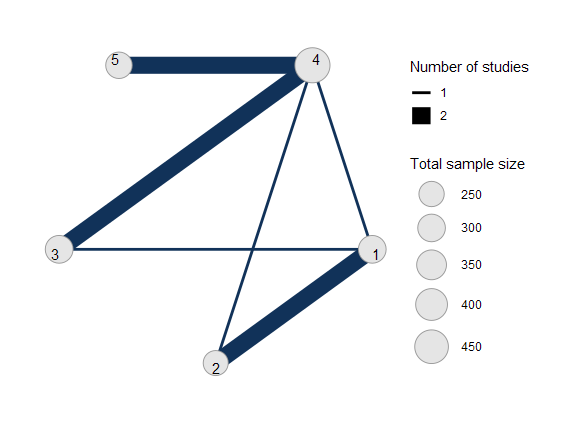### Meta-analysis models

We fit both fixed effect (FE) and random effects (RE) models.

#### Fixed effect meta-analysis

First, we fit a fixed effect model using the nma() function with trt_effects = "fixed". We use $$\mathrm{N}(0, 100^2)$$ prior distributions for the treatment effects $$d_k$$ and study-specific intercepts $$\mu_j$$. We can examine the range of parameter values implied by these prior distributions with the summary() method:

summary(normal(scale = 100))
#> A Normal prior distribution: location = 0, scale = 100.
#> 50% of the prior density lies between -67.45 and 67.45.
#> 95% of the prior density lies between -196 and 196.

The model is fitted using the nma() function.

arm_fit_FE <- nma(arm_net,
trt_effects = "fixed",
prior_intercept = normal(scale = 100),
prior_trt = normal(scale = 10))
#> Note: Setting "4" as the network reference treatment.

Basic parameter summaries are given by the print() method:

arm_fit_FE
#> A fixed effects NMA with a normal likelihood (identity link).
#> Inference for Stan model: normal.
#> 4 chains, each with iter=2000; warmup=1000; thin=1;
#> post-warmup draws per chain=1000, total post-warmup draws=4000.
#>
#>       mean se_mean   sd   2.5%   25%   50%   75% 97.5% n_eff Rhat
#> d  0.51    0.01 0.48  -0.38  0.19  0.50  0.83  1.49  1548    1
#> d -1.30    0.01 0.52  -2.29 -1.65 -1.31 -0.95 -0.26  1508    1
#> d  0.04    0.01 0.33  -0.60 -0.18  0.05  0.27  0.69  1936    1
#> d -0.30    0.00 0.21  -0.70 -0.44 -0.30 -0.16  0.10  2760    1
#> lp__ -6.70    0.06 2.35 -12.21 -8.06 -6.36 -4.97 -3.10  1544    1
#>
#> Samples were drawn using NUTS(diag_e) at Tue Dec 01 17:44:41 2020.
#> For each parameter, n_eff is a crude measure of effective sample size,
#> and Rhat is the potential scale reduction factor on split chains (at
#> convergence, Rhat=1).

By default, summaries of the study-specific intercepts $$\mu_j$$ are hidden, but could be examined by changing the pars argument:

# Not run
print(arm_fit_FE, pars = c("d", "mu"))

The prior and posterior distributions can be compared visually using the plot_prior_posterior() function:

plot_prior_posterior(arm_fit_FE)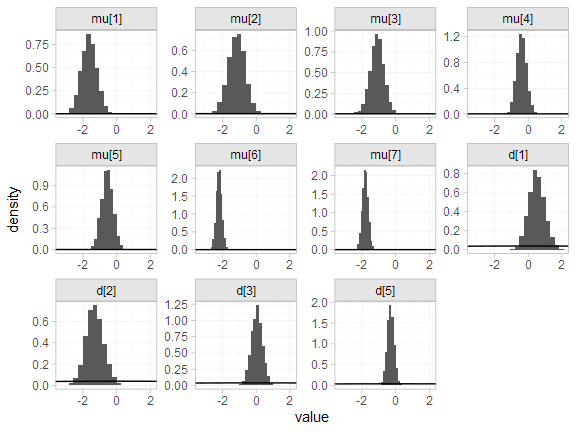#### Random effects meta-analysis

We now fit a random effects model using the nma() function with trt_effects = "random". Again, we use $$\mathrm{N}(0, 100^2)$$ prior distributions for the treatment effects $$d_k$$ and study-specific intercepts $$\mu_j$$, and we additionally use a $$\textrm{half-N}(5^2)$$ prior for the heterogeneity standard deviation $$\tau$$. We can examine the range of parameter values implied by these prior distributions with the summary() method:

summary(normal(scale = 100))
#> A Normal prior distribution: location = 0, scale = 100.
#> 50% of the prior density lies between -67.45 and 67.45.
#> 95% of the prior density lies between -196 and 196.
summary(half_normal(scale = 5))
#> A half-Normal prior distribution: location = 0, scale = 5.
#> 50% of the prior density lies between 0 and 3.37.
#> 95% of the prior density lies between 0 and 9.8.

Fitting the RE model

arm_fit_RE <- nma(arm_net,
seed = 379394727,
trt_effects = "random",
prior_intercept = normal(scale = 100),
prior_trt = normal(scale = 100),
prior_het = half_normal(scale = 5),
#> Note: Setting "4" as the network reference treatment.
#> Warning: There were 1 divergent transitions after warmup. See
#> http://mc-stan.org/misc/warnings.html#divergent-transitions-after-warmup
#> to find out why this is a problem and how to eliminate them.
#> Warning: Examine the pairs() plot to diagnose sampling problems

We do see a small number of divergent transition errors, which cannot simply be removed by increasing the value of the adapt_delta argument (by default set to 0.95 for RE models). To diagnose, we use the pairs() method to investigate where in the posterior distribution these divergences are happening (indicated by red crosses):

pairs(arm_fit_RE, pars = c("mu", "d", "delta[4: 3]", "tau"))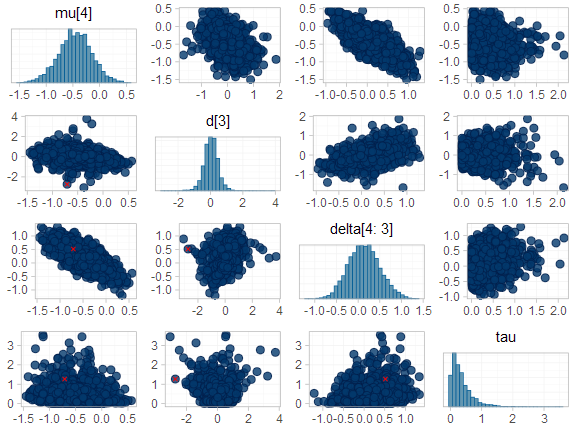The divergent transitions occur in the upper tail of the heterogeneity standard deviation. In this case, with only a small number of studies, there is not very much information to estimate the heterogeneity standard deviation and the prior distribution may be too heavy-tailed. We could consider a more informative prior distribution for the heterogeneity variance to aid estimation.

Basic parameter summaries are given by the print() method:

arm_fit_RE
#> A random effects NMA with a normal likelihood (identity link).
#> Inference for Stan model: normal.
#> 4 chains, each with iter=2000; warmup=1000; thin=1;
#> post-warmup draws per chain=1000, total post-warmup draws=4000.
#>
#>        mean se_mean   sd   2.5%    25%    50%    75% 97.5% n_eff Rhat
#> d   0.51    0.01 0.61  -0.66   0.12   0.52   0.89  1.76  1668    1
#> d  -1.32    0.02 0.68  -2.62  -1.74  -1.32  -0.90  0.00  1737    1
#> d   0.03    0.01 0.46  -0.85  -0.23   0.04   0.30  0.90  1907    1
#> d  -0.29    0.01 0.40  -1.11  -0.50  -0.29  -0.10  0.51  1991    1
#> lp__ -12.88    0.10 3.51 -20.59 -15.10 -12.55 -10.37 -6.92  1260    1
#> tau    0.36    0.01 0.37   0.01   0.12   0.27   0.49  1.34   744    1
#>
#> Samples were drawn using NUTS(diag_e) at Tue Dec 01 17:45:11 2020.
#> For each parameter, n_eff is a crude measure of effective sample size,
#> and Rhat is the potential scale reduction factor on split chains (at
#> convergence, Rhat=1).

By default, summaries of the study-specific intercepts $$\mu_j$$ and study-specific relative effects $$\delta_{jk}$$ are hidden, but could be examined by changing the pars argument:

# Not run
print(arm_fit_RE, pars = c("d", "mu", "delta"))

The prior and posterior distributions can be compared visually using the plot_prior_posterior() function:

plot_prior_posterior(arm_fit_RE)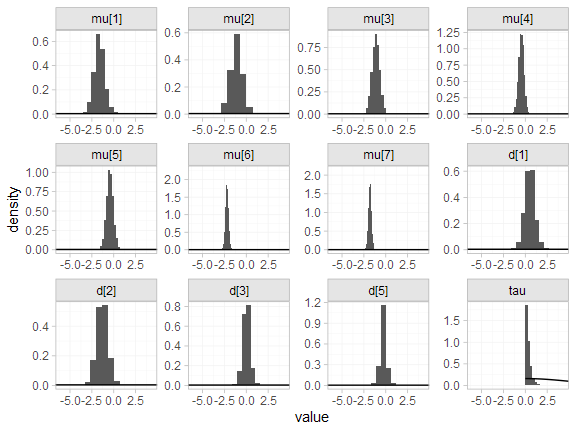#### Model comparison

Model fit can be checked using the dic() function:

(arm_dic_FE <- dic(arm_fit_FE))
#> Residual deviance: 13.4 (on 15 data points)
#>                pD: 11.1
#>               DIC: 24.4
(arm_dic_RE <- dic(arm_fit_RE))
#> Residual deviance: 13.7 (on 15 data points)
#>                pD: 12.5
#>               DIC: 26.2

Both models fit the data well, having posterior mean residual deviance close to the number of data points. The DIC is similar between models, so we choose the FE model based on parsimony.

We can also examine the residual deviance contributions with the corresponding plot() method.

plot(arm_dic_FE)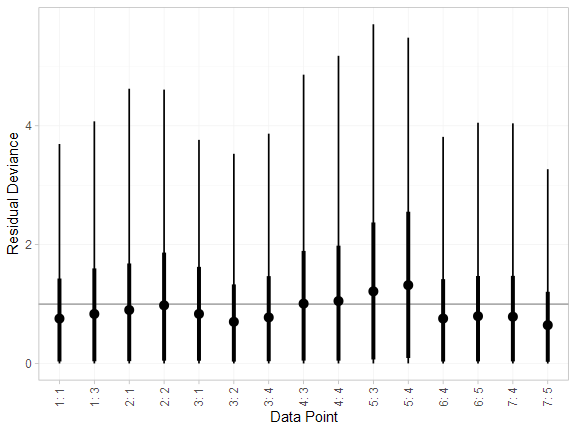plot(arm_dic_RE)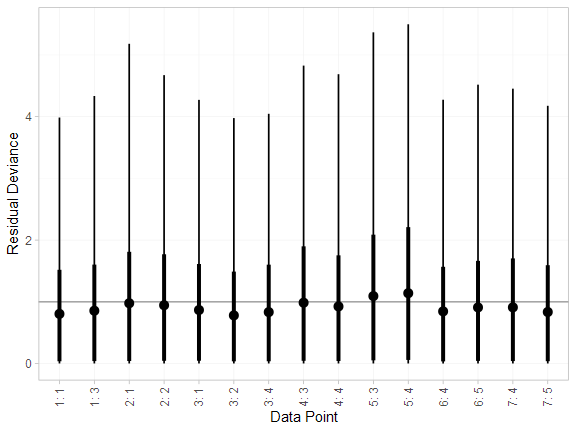### Further results

For comparison with Dias et al. (2011), we can produce relative effects against placebo using the relative_effects() function with trt_ref = 1:

(arm_releff_FE <- relative_effects(arm_fit_FE, trt_ref = 1))
#>       mean   sd  2.5%   25%   50%   75% 97.5% Bulk_ESS Tail_ESS Rhat
#> d -0.51 0.48 -1.49 -0.83 -0.50 -0.19  0.38     1575     2334    1
#> d -1.81 0.34 -2.45 -2.03 -1.82 -1.58 -1.15     6197     3170    1
#> d -0.47 0.49 -1.43 -0.80 -0.47 -0.13  0.48     2593     2912    1
#> d -0.81 0.52 -1.84 -1.16 -0.81 -0.46  0.17     1702     2512    1
plot(arm_releff_FE, ref_line = 0)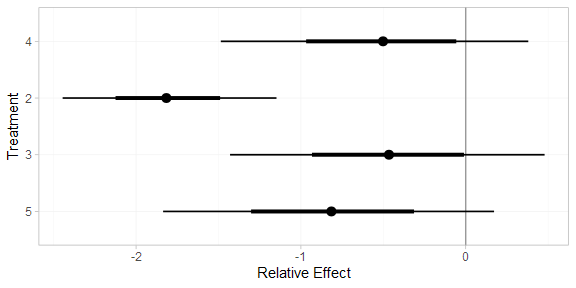(arm_releff_RE <- relative_effects(arm_fit_RE, trt_ref = 1))
#>       mean   sd  2.5%   25%   50%   75% 97.5% Bulk_ESS Tail_ESS Rhat
#> d -0.51 0.61 -1.76 -0.89 -0.52 -0.12  0.66     1687     2018    1
#> d -1.83 0.50 -2.81 -2.11 -1.82 -1.56 -0.86     3922     2156    1
#> d -0.48 0.62 -1.69 -0.86 -0.47 -0.08  0.70     2507     2535    1
#> d -0.80 0.72 -2.23 -1.24 -0.81 -0.38  0.58     1902     2024    1
plot(arm_releff_RE, ref_line = 0)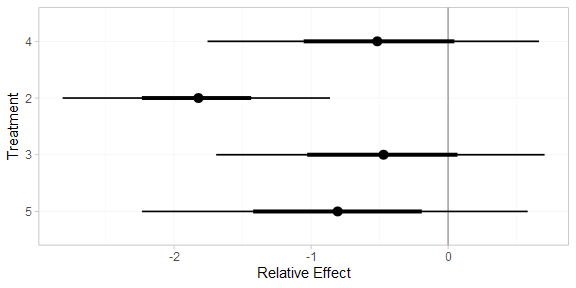Following Dias et al. (2011), we produce absolute predictions of the mean off-time reduction on each treatment assuming a Normal distribution for the outcomes on treatment 1 (placebo) with mean $$-0.73$$ and precision $$21$$. We use the predict() method, where the baseline argument takes a distr() distribution object with which we specify the corresponding Normal distribution, and we specify trt_ref = 1 to indicate that the baseline distribution corresponds to treatment 1. (Strictly speaking, type = "response" is unnecessary here, since the identity link function was used.)

arm_pred_FE <- predict(arm_fit_FE,
baseline = distr(qnorm, mean = -0.73, sd = 21^-0.5),
type = "response",
trt_ref = 1)
arm_pred_FE
#>          mean   sd  2.5%   25%   50%   75% 97.5% Bulk_ESS Tail_ESS Rhat
#> pred -1.24 0.52 -2.29 -1.59 -1.23 -0.89 -0.22     1865     2643    1
#> pred -0.73 0.22 -1.16 -0.88 -0.73 -0.58 -0.29     3856     3973    1
#> pred -2.54 0.40 -3.32 -2.81 -2.54 -2.26 -1.74     5265     3429    1
#> pred -1.20 0.53 -2.26 -1.56 -1.19 -0.84 -0.17     2831     3376    1
#> pred -1.54 0.56 -2.64 -1.92 -1.54 -1.16 -0.44     1982     2927    1
plot(arm_pred_FE)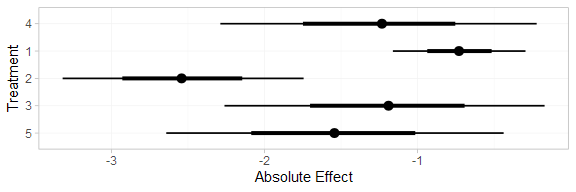arm_pred_RE <- predict(arm_fit_RE,
baseline = distr(qnorm, mean = -0.73, sd = 21^-0.5),
type = "response",
trt_ref = 1)
arm_pred_RE
#>          mean   sd  2.5%   25%   50%   75% 97.5% Bulk_ESS Tail_ESS Rhat
#> pred -1.24 0.65 -2.52 -1.65 -1.24 -0.81  0.08     1773     2073    1
#> pred -0.73 0.22 -1.16 -0.87 -0.73 -0.58 -0.30     4018     3891    1
#> pred -2.56 0.55 -3.60 -2.89 -2.56 -2.24 -1.47     3930     2192    1
#> pred -1.20 0.66 -2.47 -1.62 -1.19 -0.78  0.08     2568     2350    1
#> pred -1.53 0.75 -3.01 -2.02 -1.54 -1.06 -0.06     1983     2054    1
plot(arm_pred_RE)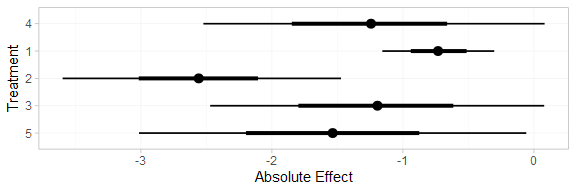If the baseline argument is omitted, predictions of mean off-time reduction will be produced for every study in the network based on their estimated baseline response $$\mu_j$$:

arm_pred_FE_studies <- predict(arm_fit_FE, type = "response")
arm_pred_FE_studies
#> ---------------------------------------------------------------------- Study: 1 ----
#>
#>             mean   sd  2.5%   25%   50%   75% 97.5% Bulk_ESS Tail_ESS Rhat
#> pred[1: 4] -1.64 0.46 -2.54 -1.96 -1.64 -1.32 -0.72     1758     2835    1
#> pred[1: 1] -1.13 0.43 -1.97 -1.43 -1.13 -0.83 -0.29     3962     3036    1
#> pred[1: 2] -2.94 0.52 -3.95 -3.29 -2.94 -2.58 -1.93     3705     3428    1
#> pred[1: 3] -1.60 0.40 -2.35 -1.86 -1.59 -1.33 -0.81     3717     3470    1
#> pred[1: 5] -1.94 0.51 -2.91 -2.29 -1.94 -1.59 -0.95     1980     3027    1
#>
#> ---------------------------------------------------------------------- Study: 2 ----
#>
#>             mean   sd  2.5%   25%   50%   75% 97.5% Bulk_ESS Tail_ESS Rhat
#> pred[2: 4] -1.16 0.50 -2.16 -1.50 -1.15 -0.81 -0.19     1500     1745    1
#> pred[2: 1] -0.64 0.26 -1.16 -0.82 -0.64 -0.47 -0.13     5356     3873    1
#> pred[2: 2] -2.45 0.25 -2.93 -2.62 -2.45 -2.28 -1.96     4984     3289    1
#> pred[2: 3] -1.11 0.52 -2.16 -1.47 -1.12 -0.75 -0.10     2423     2788    1
#> pred[2: 5] -1.46 0.54 -2.52 -1.83 -1.45 -1.08 -0.43     1609     2077    1
#>
#> ---------------------------------------------------------------------- Study: 3 ----
#>
#>             mean   sd  2.5%   25%   50%   75% 97.5% Bulk_ESS Tail_ESS Rhat
#> pred[3: 4] -1.10 0.41 -1.91 -1.38 -1.09 -0.83 -0.30     1918     2313    1
#> pred[3: 1] -0.59 0.36 -1.28 -0.83 -0.59 -0.35  0.13     4455     2993    1
#> pred[3: 2] -2.40 0.38 -3.14 -2.65 -2.40 -2.15 -1.65     4202     2959    1
#> pred[3: 3] -1.06 0.47 -1.97 -1.38 -1.06 -0.74 -0.16     3024     3121    1
#> pred[3: 5] -1.40 0.46 -2.29 -1.72 -1.41 -1.10 -0.50     2126     2827    1
#>
#> ---------------------------------------------------------------------- Study: 4 ----
#>
#>             mean   sd  2.5%   25%   50%   75% 97.5% Bulk_ESS Tail_ESS Rhat
#> pred[4: 4] -0.40 0.31 -0.99 -0.60 -0.40 -0.19  0.21     2289     2736    1
#> pred[4: 1]  0.12 0.51 -0.86 -0.23  0.11  0.45  1.14     2418     2986    1
#> pred[4: 2] -1.69 0.55 -2.77 -2.07 -1.70 -1.32 -0.58     2285     3135    1
#> pred[4: 3] -0.35 0.24 -0.82 -0.52 -0.35 -0.19  0.12     5384     3528    1
#> pred[4: 5] -0.70 0.37 -1.43 -0.96 -0.70 -0.45  0.04     2596     2885    1
#>
#> ---------------------------------------------------------------------- Study: 5 ----
#>
#>             mean   sd  2.5%   25%   50%   75% 97.5% Bulk_ESS Tail_ESS Rhat
#> pred[5: 4] -0.55 0.34 -1.21 -0.78 -0.55 -0.32  0.13     2736     2575    1
#> pred[5: 1] -0.04 0.53 -1.07 -0.40 -0.05  0.33  1.01     2471     2583    1
#> pred[5: 2] -1.85 0.57 -2.94 -2.24 -1.85 -1.46 -0.70     2221     2873    1
#> pred[5: 3] -0.51 0.30 -1.09 -0.71 -0.51 -0.31  0.07     5073     3153    1
#> pred[5: 5] -0.85 0.40 -1.67 -1.12 -0.85 -0.59 -0.06     2801     3042    1
#>
#> ---------------------------------------------------------------------- Study: 6 ----
#>
#>             mean   sd  2.5%   25%   50%   75% 97.5% Bulk_ESS Tail_ESS Rhat
#> pred[6: 4] -2.20 0.17 -2.54 -2.31 -2.20 -2.08 -1.86     2909     2732    1
#> pred[6: 1] -1.69 0.51 -2.65 -2.03 -1.69 -1.36 -0.68     1690     2563    1
#> pred[6: 2] -3.49 0.54 -4.52 -3.87 -3.50 -3.13 -2.41     1625     2472    1
#> pred[6: 3] -2.16 0.37 -2.89 -2.41 -2.16 -1.91 -1.42     2194     2597    1
#> pred[6: 5] -2.50 0.17 -2.84 -2.61 -2.50 -2.39 -2.16     6141     3063    1
#>
#> ---------------------------------------------------------------------- Study: 7 ----
#>
#>             mean   sd  2.5%   25%   50%   75% 97.5% Bulk_ESS Tail_ESS Rhat
#> pred[7: 4] -1.80 0.18 -2.15 -1.92 -1.80 -1.68 -1.46     3187     2733    1
#> pred[7: 1] -1.29 0.51 -2.27 -1.64 -1.28 -0.95 -0.27     1670     2186    1
#> pred[7: 2] -3.09 0.55 -4.12 -3.47 -3.10 -2.73 -2.00     1601     2325    1
#> pred[7: 3] -1.76 0.37 -2.46 -2.01 -1.76 -1.50 -1.04     2352     2343    1
#> pred[7: 5] -2.10 0.20 -2.50 -2.24 -2.10 -1.97 -1.70     4750     3194    1
plot(arm_pred_FE_studies)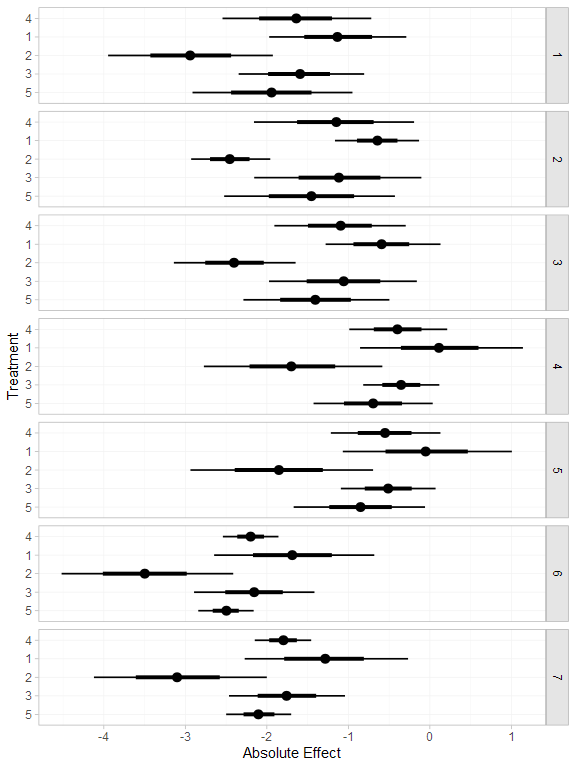We can also produce treatment rankings, rank probabilities, and cumulative rank probabilities.

(arm_ranks <- posterior_ranks(arm_fit_FE))
#>         mean   sd 2.5% 25% 50% 75% 97.5% Bulk_ESS Tail_ESS Rhat
#> rank 3.50 0.72    2   3   3   4     5     1847       NA    1
#> rank 4.64 0.76    2   5   5   5     5     2408       NA    1
#> rank 1.05 0.28    1   1   1   1     2     2761     2789    1
#> rank 3.53 0.93    2   3   4   4     5     2870       NA    1
#> rank 2.28 0.67    1   2   2   2     4     2878     2993    1
plot(arm_ranks)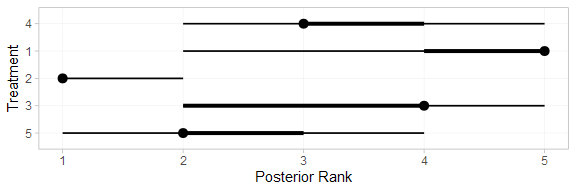(arm_rankprobs <- posterior_rank_probs(arm_fit_FE))
#>      p_rank p_rank p_rank p_rank p_rank
#> d      0.00      0.04      0.49      0.38      0.09
#> d      0.00      0.04      0.07      0.12      0.78
#> d      0.96      0.03      0.01      0.00      0.00
#> d      0.00      0.17      0.25      0.45      0.13
#> d      0.04      0.72      0.18      0.05      0.01
plot(arm_rankprobs)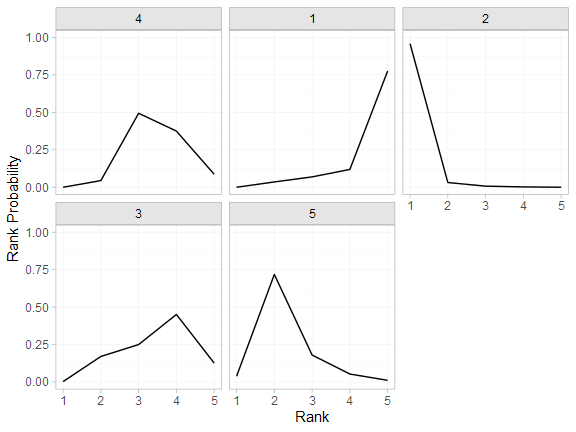(arm_cumrankprobs <- posterior_rank_probs(arm_fit_FE, cumulative = TRUE))
#>      p_rank p_rank p_rank p_rank p_rank
#> d      0.00      0.04      0.54      0.91         1
#> d      0.00      0.04      0.10      0.22         1
#> d      0.96      0.99      1.00      1.00         1
#> d      0.00      0.17      0.42      0.87         1
#> d      0.04      0.76      0.94      0.99         1
plot(arm_cumrankprobs)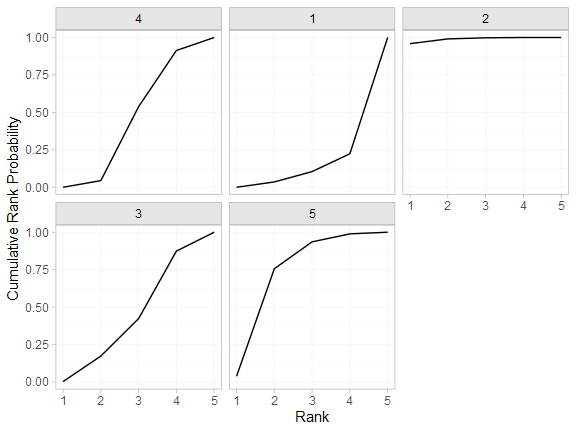## Analysis of contrast-based data

We now perform an analysis using the contrast-based data (mean differences and standard errors).

### Setting up the network

With contrast-level data giving the mean difference in off-time reduction (diff) and standard error (se_diff), we use the function set_agd_contrast() to set up the network.

contr_net <- set_agd_contrast(parkinsons,
study = studyn,
trt = trtn,
y = diff,
se = se_diff,
sample_size = n)
contr_net
#> A network with 7 AgD studies (contrast-based).
#>
#> -------------------------------------------------- AgD studies (contrast-based) ----
#>  Study Treatments
#>  1     2: 1 | 3
#>  2     2: 1 | 2
#>  3     3: 1 | 2 | 4
#>  4     2: 3 | 4
#>  5     2: 3 | 4
#>  6     2: 4 | 5
#>  7     2: 4 | 5
#>
#>  Outcome type: continuous
#> ------------------------------------------------------------------------------------
#> Total number of treatments: 5
#> Total number of studies: 7
#> Reference treatment is: 4
#> Network is connected

The sample_size argument is optional, but enables the nodes to be weighted by sample size in the network plot.

Plot the network structure.

plot(contr_net, weight_edges = TRUE, weight_nodes = TRUE)### Meta-analysis models

We fit both fixed effect (FE) and random effects (RE) models.

#### Fixed effect meta-analysis

First, we fit a fixed effect model using the nma() function with trt_effects = "fixed". We use $$\mathrm{N}(0, 100^2)$$ prior distributions for the treatment effects $$d_k$$. We can examine the range of parameter values implied by these prior distributions with the summary() method:

summary(normal(scale = 100))
#> A Normal prior distribution: location = 0, scale = 100.
#> 50% of the prior density lies between -67.45 and 67.45.
#> 95% of the prior density lies between -196 and 196.

The model is fitted using the nma() function.

contr_fit_FE <- nma(contr_net,
trt_effects = "fixed",
prior_trt = normal(scale = 100))
#> Note: Setting "4" as the network reference treatment.

Basic parameter summaries are given by the print() method:

contr_fit_FE
#> A fixed effects NMA with a normal likelihood (identity link).
#> Inference for Stan model: normal.
#> 4 chains, each with iter=2000; warmup=1000; thin=1;
#> post-warmup draws per chain=1000, total post-warmup draws=4000.
#>
#>       mean se_mean   sd  2.5%   25%   50%   75% 97.5% n_eff Rhat
#> d  0.52    0.01 0.47 -0.38  0.20  0.51  0.83  1.44  2252    1
#> d -1.29    0.01 0.51 -2.26 -1.65 -1.29 -0.95 -0.31  2333    1
#> d  0.03    0.01 0.33 -0.59 -0.19  0.03  0.26  0.67  2612    1
#> d -0.30    0.00 0.21 -0.71 -0.44 -0.30 -0.16  0.11  3590    1
#> lp__ -3.16    0.04 1.44 -6.65 -3.91 -2.81 -2.10 -1.38  1679    1
#>
#> Samples were drawn using NUTS(diag_e) at Tue Dec 01 17:45:57 2020.
#> For each parameter, n_eff is a crude measure of effective sample size,
#> and Rhat is the potential scale reduction factor on split chains (at
#> convergence, Rhat=1).

The prior and posterior distributions can be compared visually using the plot_prior_posterior() function:

plot_prior_posterior(contr_fit_FE)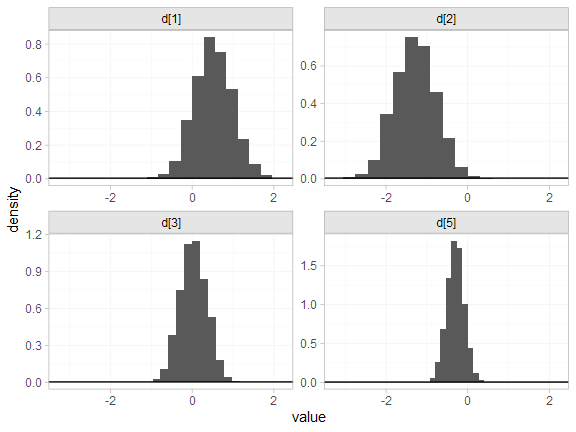#### Random effects meta-analysis

We now fit a random effects model using the nma() function with trt_effects = "random". Again, we use $$\mathrm{N}(0, 100^2)$$ prior distributions for the treatment effects $$d_k$$, and we additionally use a $$\textrm{half-N}(5^2)$$ prior for the heterogeneity standard deviation $$\tau$$. We can examine the range of parameter values implied by these prior distributions with the summary() method:

summary(normal(scale = 100))
#> A Normal prior distribution: location = 0, scale = 100.
#> 50% of the prior density lies between -67.45 and 67.45.
#> 95% of the prior density lies between -196 and 196.
summary(half_normal(scale = 5))
#> A half-Normal prior distribution: location = 0, scale = 5.
#> 50% of the prior density lies between 0 and 3.37.
#> 95% of the prior density lies between 0 and 9.8.

Fitting the RE model

contr_fit_RE <- nma(contr_net,
seed = 1150676438,
trt_effects = "random",
prior_trt = normal(scale = 100),
prior_het = half_normal(scale = 5),
#> Note: Setting "4" as the network reference treatment.
#> Warning: There were 6 divergent transitions after warmup. See
#> http://mc-stan.org/misc/warnings.html#divergent-transitions-after-warmup
#> to find out why this is a problem and how to eliminate them.
#> Warning: Examine the pairs() plot to diagnose sampling problems

We do see a small number of divergent transition errors, which cannot simply be removed by increasing the value of the adapt_delta argument (by default set to 0.95 for RE models). To diagnose, we use the pairs() method to investigate where in the posterior distribution these divergences are happening (indicated by red crosses):

pairs(contr_fit_RE, pars = c("d", "delta[4: 4 vs. 3]", "tau"))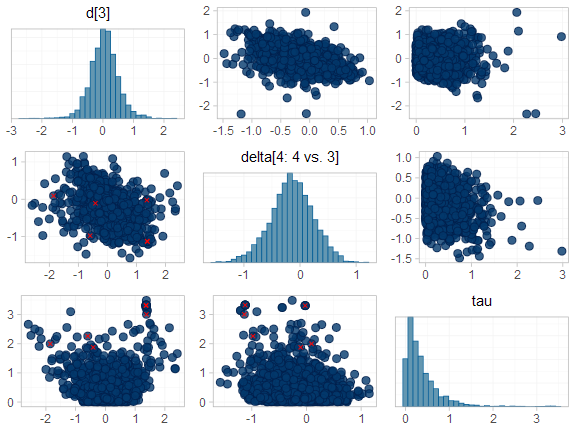The divergent transitions occur in the upper tail of the heterogeneity standard deviation. In this case, with only a small number of studies, there is not very much information to estimate the heterogeneity standard deviation and the prior distribution may be too heavy-tailed. We could consider a more informative prior distribution for the heterogeneity variance to aid estimation.

Basic parameter summaries are given by the print() method:

contr_fit_RE
#> A random effects NMA with a normal likelihood (identity link).
#> Inference for Stan model: normal.
#> 4 chains, each with iter=2000; warmup=1000; thin=1;
#> post-warmup draws per chain=1000, total post-warmup draws=4000.
#>
#>       mean se_mean   sd   2.5%    25%   50%   75% 97.5% n_eff Rhat
#> d  0.55    0.02 0.64  -0.67   0.18  0.56  0.93  1.74  1440 1.00
#> d -1.29    0.02 0.77  -2.68  -1.71 -1.29 -0.86  0.12  1037 1.01
#> d  0.04    0.01 0.48  -0.88  -0.24  0.04  0.32  1.02  1691 1.00
#> d -0.28    0.02 0.46  -1.12  -0.50 -0.30 -0.09  0.62   790 1.00
#> lp__ -8.39    0.08 2.87 -14.65 -10.19 -8.12 -6.31 -3.55  1265 1.00
#> tau   0.39    0.02 0.42   0.01   0.12  0.27  0.51  1.46   326 1.01
#>
#> Samples were drawn using NUTS(diag_e) at Tue Dec 01 17:46:22 2020.
#> For each parameter, n_eff is a crude measure of effective sample size,
#> and Rhat is the potential scale reduction factor on split chains (at
#> convergence, Rhat=1).

By default, summaries of the study-specific relative effects $$\delta_{jk}$$ are hidden, but could be examined by changing the pars argument:

# Not run
print(contr_fit_RE, pars = c("d", "delta"))

The prior and posterior distributions can be compared visually using the plot_prior_posterior() function:

plot_prior_posterior(contr_fit_RE)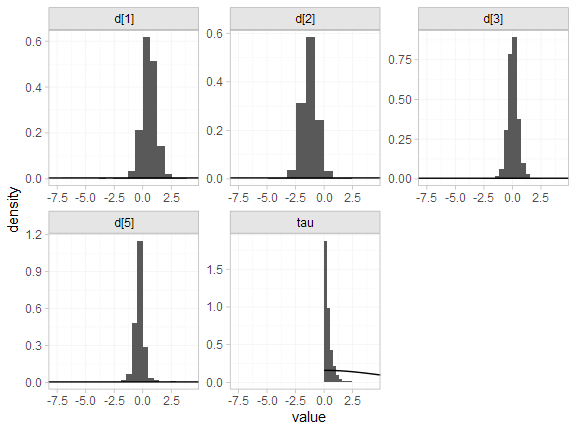#### Model comparison

Model fit can be checked using the dic() function:

(contr_dic_FE <- dic(contr_fit_FE))
#> Residual deviance: 6.3 (on 8 data points)
#>                pD: 4
#>               DIC: 10.4
(contr_dic_RE <- dic(contr_fit_RE))
#> Residual deviance: 6.7 (on 8 data points)
#>                pD: 5.5
#>               DIC: 12.2

Both models fit the data well, having posterior mean residual deviance close to the number of data points. The DIC is similar between models, so we choose the FE model based on parsimony.

We can also examine the residual deviance contributions with the corresponding plot() method.

plot(contr_dic_FE)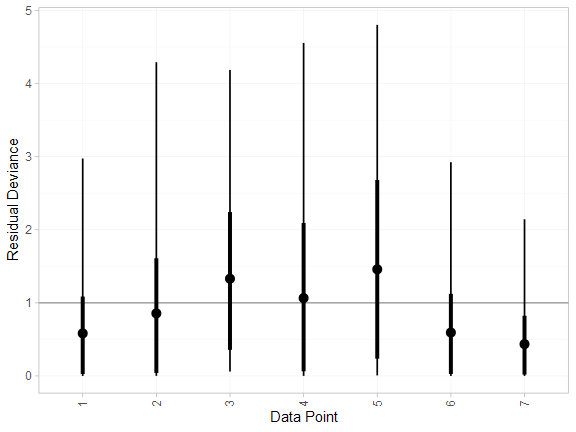plot(contr_dic_RE)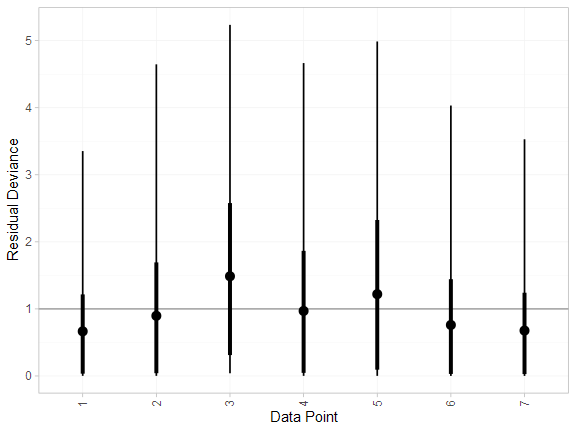### Further results

For comparison with Dias et al. (2011), we can produce relative effects against placebo using the relative_effects() function with trt_ref = 1:

(contr_releff_FE <- relative_effects(contr_fit_FE, trt_ref = 1))
#>       mean   sd  2.5%   25%   50%   75% 97.5% Bulk_ESS Tail_ESS Rhat
#> d -0.52 0.47 -1.44 -0.83 -0.51 -0.20  0.38     2257     2790    1
#> d -1.81 0.34 -2.47 -2.04 -1.81 -1.58 -1.15     5091     3026    1
#> d -0.48 0.48 -1.43 -0.81 -0.48 -0.16  0.46     3321     2786    1
#> d -0.81 0.51 -1.83 -1.15 -0.81 -0.48  0.17     2417     2625    1
plot(contr_releff_FE, ref_line = 0)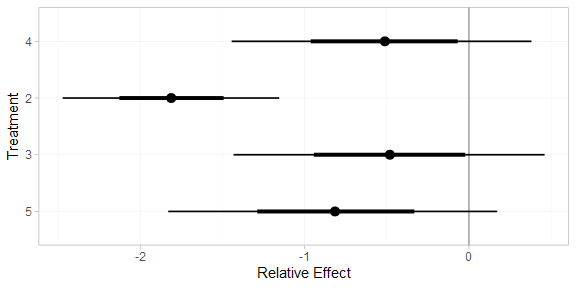(contr_releff_RE <- relative_effects(contr_fit_RE, trt_ref = 1))
#>       mean   sd  2.5%   25%   50%   75% 97.5% Bulk_ESS Tail_ESS Rhat
#> d -0.55 0.64 -1.74 -0.93 -0.56 -0.18  0.67     1701     1250    1
#> d -1.84 0.58 -2.88 -2.14 -1.84 -1.56 -0.84     2601     1492    1
#> d -0.51 0.66 -1.81 -0.91 -0.50 -0.12  0.77     2094     1349    1
#> d -0.83 0.83 -2.34 -1.29 -0.86 -0.41  0.72     1561     1085    1
plot(contr_releff_RE, ref_line = 0)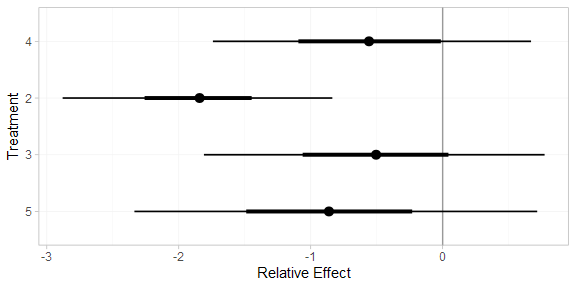Following Dias et al. (2011), we produce absolute predictions of the mean off-time reduction on each treatment assuming a Normal distribution for the outcomes on treatment 1 (placebo) with mean $$-0.73$$ and precision $$21$$. We use the predict() method, where the baseline argument takes a distr() distribution object with which we specify the corresponding Normal distribution, and we specify trt_ref = 1 to indicate that the baseline distribution corresponds to treatment 1. (Strictly speaking, type = "response" is unnecessary here, since the identity link function was used.)

contr_pred_FE <- predict(contr_fit_FE,
baseline = distr(qnorm, mean = -0.73, sd = 21^-0.5),
type = "response",
trt_ref = 1)
contr_pred_FE
#>          mean   sd  2.5%   25%   50%   75% 97.5% Bulk_ESS Tail_ESS Rhat
#> pred -1.25 0.52 -2.24 -1.60 -1.25 -0.90 -0.24     2697     2872    1
#> pred -0.73 0.22 -1.16 -0.88 -0.73 -0.58 -0.30     3967     3603    1
#> pred -2.54 0.41 -3.35 -2.81 -2.55 -2.26 -1.74     5041     3796    1
#> pred -1.21 0.53 -2.26 -1.56 -1.22 -0.86 -0.16     3512     3475    1
#> pred -1.54 0.55 -2.60 -1.92 -1.55 -1.17 -0.44     2789     2963    1
plot(contr_pred_FE)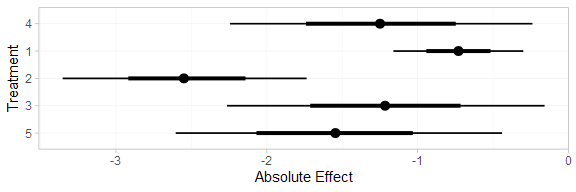contr_pred_RE <- predict(contr_fit_RE,
baseline = distr(qnorm, mean = -0.73, sd = 21^-0.5),
type = "response",
trt_ref = 1)
contr_pred_RE
#>          mean   sd  2.5%   25%   50%   75% 97.5% Bulk_ESS Tail_ESS Rhat
#> pred -1.29 0.67 -2.56 -1.69 -1.29 -0.89 -0.01     1758     1890    1
#> pred -0.73 0.22 -1.16 -0.88 -0.73 -0.59 -0.30     3676     3583    1
#> pred -2.58 0.62 -3.68 -2.91 -2.59 -2.26 -1.49     2726     1752    1
#> pred -1.24 0.69 -2.63 -1.67 -1.25 -0.81  0.09     2052     1410    1
#> pred -1.57 0.85 -3.12 -2.05 -1.60 -1.13  0.01     1594     1168    1
plot(contr_pred_RE)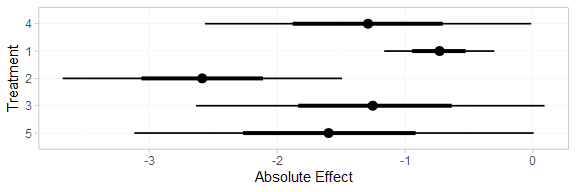If the baseline argument is omitted an error will be raised, as there are no study baselines estimated in this network.

# Not run
predict(contr_fit_FE, type = "response")

We can also produce treatment rankings, rank probabilities, and cumulative rank probabilities.

(contr_ranks <- posterior_ranks(contr_fit_FE))
#>         mean   sd 2.5% 25% 50% 75% 97.5% Bulk_ESS Tail_ESS Rhat
#> rank 3.52 0.71    2   3   3   4     5     2470       NA    1
#> rank 4.65 0.76    2   5   5   5     5     2754       NA    1
#> rank 1.05 0.26    1   1   1   1     2     2656     2693    1
#> rank 3.49 0.93    2   3   4   4     5     3384       NA    1
#> rank 2.30 0.66    1   2   2   3     4     2740     2717    1
plot(contr_ranks)(contr_rankprobs <- posterior_rank_probs(contr_fit_FE))
#>      p_rank p_rank p_rank p_rank p_rank
#> d      0.00      0.04      0.48      0.39      0.08
#> d      0.00      0.04      0.06      0.12      0.79
#> d      0.96      0.03      0.00      0.00      0.00
#> d      0.00      0.18      0.26      0.44      0.12
#> d      0.03      0.71      0.19      0.05      0.01
plot(contr_rankprobs)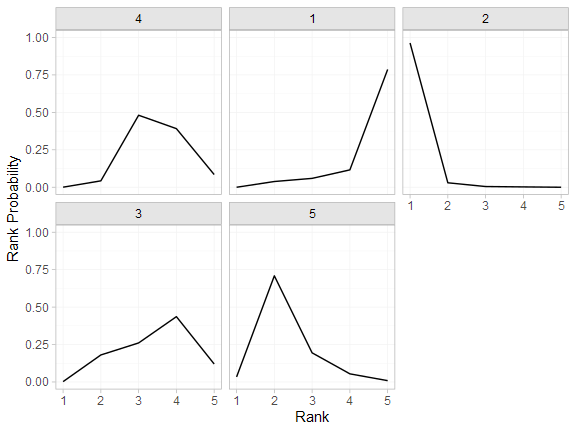(contr_cumrankprobs <- posterior_rank_probs(contr_fit_FE, cumulative = TRUE))
#>      p_rank p_rank p_rank p_rank p_rank
#> d      0.00      0.04      0.52      0.92         1
#> d      0.00      0.04      0.10      0.21         1
#> d      0.96      0.99      1.00      1.00         1
#> d      0.00      0.18      0.44      0.88         1
#> d      0.03      0.74      0.94      0.99         1
plot(contr_cumrankprobs)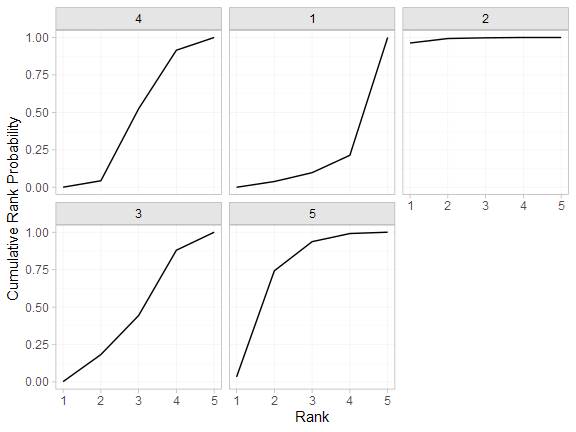## Analysis of mixed arm-based and contrast-based data

We now perform an analysis where some studies contribute arm-based data, and other contribute contrast-based data. Replicating Dias et al. (2011), we consider arm-based data from studies 1-3, and contrast-based data from studies 4-7.

studies <- parkinsons\$studyn
(parkinsons_arm <- parkinsons[studies %in% 1:3, ])
#>   studyn trtn     y    se   n  diff se_diff
#> 1      1    1 -1.22 0.504  54    NA   0.504
#> 2      1    3 -1.53 0.439  95 -0.31   0.668
#> 3      2    1 -0.70 0.282 172    NA   0.282
#> 4      2    2 -2.40 0.258 173 -1.70   0.382
#> 5      3    1 -0.30 0.505  76    NA   0.505
#> 6      3    2 -2.60 0.510  71 -2.30   0.718
#> 7      3    4 -1.20 0.478  81 -0.90   0.695
(parkinsons_contr <- parkinsons[studies %in% 4:7, ])
#>    studyn trtn     y    se   n  diff se_diff
#> 8       4    3 -0.24 0.265 128    NA   0.265
#> 9       4    4 -0.59 0.354  72 -0.35   0.442
#> 10      5    3 -0.73 0.335  80    NA   0.335
#> 11      5    4 -0.18 0.442  46  0.55   0.555
#> 12      6    4 -2.20 0.197 137    NA   0.197
#> 13      6    5 -2.50 0.190 131 -0.30   0.274
#> 14      7    4 -1.80 0.200 154    NA   0.200
#> 15      7    5 -2.10 0.250 143 -0.30   0.320

### Setting up the network

We use the functions set_agd_arm() and set_agd_contrast() to set up the respective data sources within the network, and then combine together with combine_network().

mix_arm_net <- set_agd_arm(parkinsons_arm,
study = studyn,
trt = trtn,
y = y,
se = se,
sample_size = n)

mix_contr_net <- set_agd_contrast(parkinsons_contr,
study = studyn,
trt = trtn,
y = diff,
se = se_diff,
sample_size = n)

mix_net <- combine_network(mix_arm_net, mix_contr_net)
mix_net
#> A network with 3 AgD studies (arm-based), and 4 AgD studies (contrast-based).
#>
#> ------------------------------------------------------- AgD studies (arm-based) ----
#>  Study Treatments
#>  1     2: 1 | 3
#>  2     2: 1 | 2
#>  3     3: 1 | 2 | 4
#>
#>  Outcome type: continuous
#> -------------------------------------------------- AgD studies (contrast-based) ----
#>  Study Treatments
#>  4     2: 3 | 4
#>  5     2: 3 | 4
#>  6     2: 4 | 5
#>  7     2: 4 | 5
#>
#>  Outcome type: continuous
#> ------------------------------------------------------------------------------------
#> Total number of treatments: 5
#> Total number of studies: 7
#> Reference treatment is: 4
#> Network is connected

The sample_size argument is optional, but enables the nodes to be weighted by sample size in the network plot.

Plot the network structure.

plot(mix_net, weight_edges = TRUE, weight_nodes = TRUE)### Meta-analysis models

We fit both fixed effect (FE) and random effects (RE) models.

#### Fixed effect meta-analysis

First, we fit a fixed effect model using the nma() function with trt_effects = "fixed". We use $$\mathrm{N}(0, 100^2)$$ prior distributions for the treatment effects $$d_k$$ and study-specific intercepts $$\mu_j$$. We can examine the range of parameter values implied by these prior distributions with the summary() method:

summary(normal(scale = 100))
#> A Normal prior distribution: location = 0, scale = 100.
#> 50% of the prior density lies between -67.45 and 67.45.
#> 95% of the prior density lies between -196 and 196.

The model is fitted using the nma() function.

mix_fit_FE <- nma(mix_net,
trt_effects = "fixed",
prior_intercept = normal(scale = 100),
prior_trt = normal(scale = 100))
#> Note: Setting "4" as the network reference treatment.

Basic parameter summaries are given by the print() method:

mix_fit_FE
#> A fixed effects NMA with a normal likelihood (identity link).
#> Inference for Stan model: normal.
#> 4 chains, each with iter=2000; warmup=1000; thin=1;
#> post-warmup draws per chain=1000, total post-warmup draws=4000.
#>
#>       mean se_mean   sd  2.5%   25%   50%   75% 97.5% n_eff Rhat
#> d  0.52    0.01 0.49 -0.44  0.21  0.52  0.84  1.47  1337    1
#> d -1.28    0.01 0.53 -2.33 -1.65 -1.28 -0.94 -0.26  1379    1
#> d  0.05    0.01 0.32 -0.59 -0.17  0.05  0.26  0.65  2284    1
#> d -0.30    0.00 0.21 -0.70 -0.44 -0.30 -0.16  0.09  2798    1
#> lp__ -4.59    0.05 1.91 -9.26 -5.54 -4.24 -3.20 -1.98  1715    1
#>
#> Samples were drawn using NUTS(diag_e) at Tue Dec 01 17:46:53 2020.
#> For each parameter, n_eff is a crude measure of effective sample size,
#> and Rhat is the potential scale reduction factor on split chains (at
#> convergence, Rhat=1).

By default, summaries of the study-specific intercepts $$\mu_j$$ are hidden, but could be examined by changing the pars argument:

# Not run
print(mix_fit_FE, pars = c("d", "mu"))

The prior and posterior distributions can be compared visually using the plot_prior_posterior() function:

plot_prior_posterior(mix_fit_FE)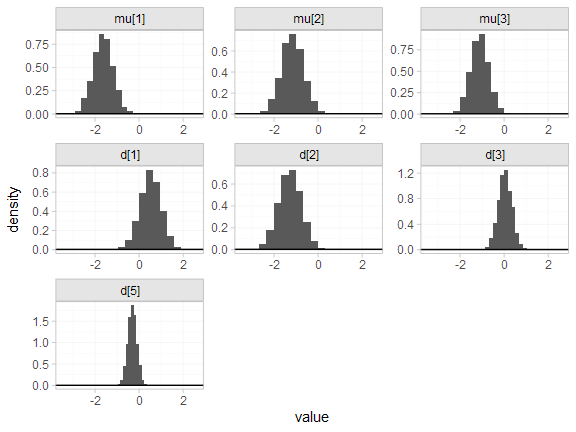#### Random effects meta-analysis

We now fit a random effects model using the nma() function with trt_effects = "random". Again, we use $$\mathrm{N}(0, 100^2)$$ prior distributions for the treatment effects $$d_k$$ and study-specific intercepts $$\mu_j$$, and we additionally use a $$\textrm{half-N}(5^2)$$ prior for the heterogeneity standard deviation $$\tau$$. We can examine the range of parameter values implied by these prior distributions with the summary() method:

summary(normal(scale = 100))
#> A Normal prior distribution: location = 0, scale = 100.
#> 50% of the prior density lies between -67.45 and 67.45.
#> 95% of the prior density lies between -196 and 196.
summary(half_normal(scale = 5))
#> A half-Normal prior distribution: location = 0, scale = 5.
#> 50% of the prior density lies between 0 and 3.37.
#> 95% of the prior density lies between 0 and 9.8.

Fitting the RE model

mix_fit_RE <- nma(mix_net,
seed = 437219664,
trt_effects = "random",
prior_intercept = normal(scale = 100),
prior_trt = normal(scale = 100),
prior_het = half_normal(scale = 5),
#> Note: Setting "4" as the network reference treatment.
We do see a small number of divergent transition errors, which cannot simply be removed by increasing the value of the adapt_delta argument (by default set to 0.95 for RE models). To diagnose, we use the pairs() method to investigate where in the posterior distribution these divergences are happening (indicated by red crosses):
pairs(mix_fit_RE, pars = c("d", "delta[4: 4 vs. 3]", "tau"))